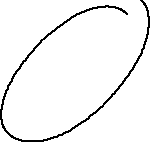NAME

Prima::Drawable::Path - stroke and fill complex paths

DESCRIPTION

The module augments the `Prima::Drawable` drawing and plotting functionality by implementing paths that allow arbitrary combination of polylines, splines, and arcs, to be used for drawing or clipping shapes.

SYNOPSIS

``````        # draws elliptic spiral
my ( \$d1, \$dx ) = ( 0.8, 0.05 );
\$canvas-> new_path->
scale(200, 100)->
rotate(45)->
arc( 0, 0, \$d1 + \$dx * 0, \$d1 + \$dx * 1, 0, 90)->
arc( 0, 0, \$d1 + \$dx * 2, \$d1 + \$dx * 1, 90, 180)->
arc( 0, 0, \$d1 + \$dx * 2, \$d1 + \$dx * 3, 180, 270)->
arc( 0, 0, \$d1 + \$dx * 4, \$d1 + \$dx * 3, 270, 360)->
stroke;``````API

Primitives

All primitives come in two versions, with absolute and relative coordinates. The absolute version draws a graphic primitive so that its starting point (or a reference point) is at (0,0). The relative version, called with an 'r' (f.ex. `line` vs `rline`) has its starting point as the ending point of the previous primitive (or (0,0) if there's none).

arc CENTER_X, CENTER_Y, DIAMETER_X, DIAMETER_Y, ANGLE_START, ANGLE_END, TILT = 0

Adds elliptic arc to path centered around (CENTER_X,CENTER_Y).

circular_arc ANGLE_START, ANGLE_END

Adds circular arc to the path. Note that adding transformations will effectively make it into elliptic arc, which is used internally by `arc` and `rarc`.

ellipse CENTER_X, CENTER_Y, DIAMETER_X, DIAMETER_Y = DIAMETER_X, TILT = 0

Adds full ellipse to the path.

line, rline @POINTS

rarc DIAMETER_X, DIAMETER_Y, ANGLE_START, ANGLE_END, TILT = 0

Adds elliptic arc to path so that the first point of the arc starts on the last point of the previous primitive, or (0,0) if there's none.

spline, rspline \$POINTS, %OPTIONS.

Adds B-spline to path. See "spline" in Prima::Drawable for `%OPTIONS` descriptions.

Transformations

Transformation calls change the current path properties (matrix etc) so that all subsequent calls will use them until a call to `restore` is used. `save` and `restore` implement a stacking mechanism, so that local transformations can be made.

The final transformations calculate coordinates the new and the existing matrices:

``  P' = NewMatrix * P``
matrix A, B, C, D, Tx, Ty

Applies transformation matrix to the path. The matrix, as used by the module, is formed as such:

``````  A  B  0
C  D  0
Tx Ty 1``````

and when applied to 2D coordinates, is calculated as

``````  X' = AX + CY + Tx
Y' = BX + DY + Ty``````
precision INTEGER

Selects current precision for splines and arcs. See "spline" in Prima::Drawable, `precision` entry.

restore

Pops the stack entry and replaces the current matrix and graphic properties with it.

rotate ANGLE

Adds rotation to the current matrix

save

Duplicates the current matrix and graphic properties and pushes them to the stack.

shear X, Y = X

Adds shearing to the current matrix

scale X, Y = X

Adds scaling to the current matrix

translate X, Y = X

Adds offset to the current matrix

Operations

These methods perform actual path rendering, that was delayed until that, and will create an array of points that can be used for actual drawing.

clip %options

Returns 1-bit image with clipping mask of the path. `%options` can be used to pass `fillWinding` property that affects the result of the filled shape.

extents

Returns 2 points that box the path.

points

Runs all accumulated commands, and returns rendered set of points, suitable for further calls to `Prima::Drawable::polyline` and `Prima::Drawable::fillpoly`.

last_matrix

Return CTM resulted after running all commands

fill

Paints a filled shape over the path

stroke

Draws a polyline over the path

region WINDING=0

Creates a region object from polygonal shape. If WINDING is set, applies fill winding mode (see "fillWinding" in Drawable for more).

Methods for custom primitives

append PATH

Copies all commands from another PATH object. The PATH object doesn't need to have balanced stacking brackets `save` and `restore`, and can be viewed as a macro.

identity

Returns identity matrix

matrix_apply @POINTS

Applies current matrix to POINTS, returns the transformed points. If @POINTS is a list, returns list; if it is an array reference, returns array reference.

AUTHOR

Dmitry Karasik, <dmitry@karasik.eu.org>.